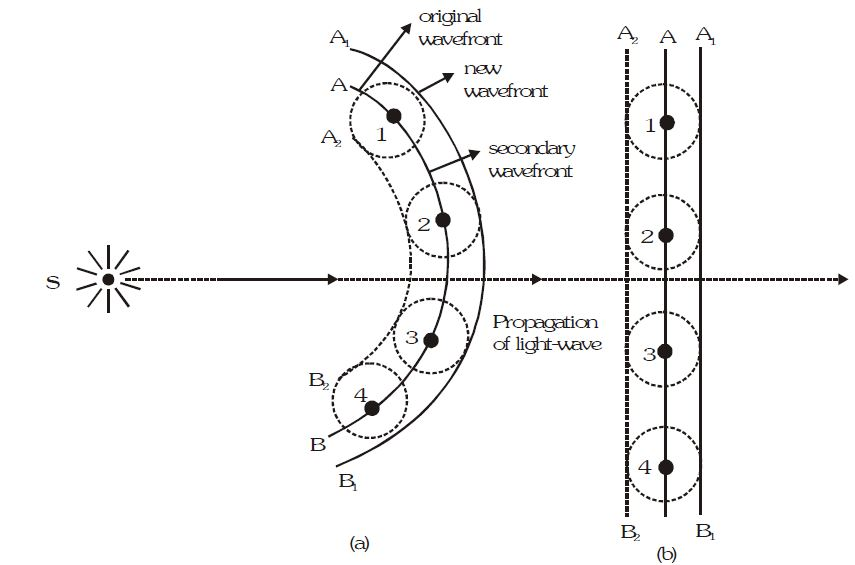# Wave Theory of Light

LIGHT

The physical cause, with the help of which our eyes experience the sensation of vision, is known as light or the form of energy, which excites our retina and produce the sensation of vision, is known as light.

PROPERTIES OF VISIBLE LIGHT

• No material medium is required for the propagation of light energy i.e. it travels even in vacuum.

• Its velocity is constant in all inertial frames i.e. it is an absolute constant. It is independent of the relative velocity between source and the observer.

• Its velocity in vacuum is maximum whose value is 3 × 10^8 m/s.

• It lies in the visible region of electromagnetic spectrum whose wavelength range is from 4000 Å to 8000 Å.

• Its energy is of the order of eV.

• It propagates in straight line.

• It exhibits the phenomena of reflection, refraction, interference, diffraction, polarisation and double refraction.

• It can emit electrons from metal surface i.e. it can produce photoelectric effect.

• It produces thermal effect and exerts pressure when incident upon a surface. It proves that light has momentum and energy.

• Its velocity is different in different media. In rarer medium it is more and in denser medium it is less.

• Light energy propagates via two processes.

(a) The particles of the medium carry energy from one point of the medium to another.

(b) The particles transmit energy to the neighbouring particles and in this way energy propagates in the form of a disturbance.

DIFFERENT THEORIES OF LIGHT

• Newton’s corpuscular theory of light.

• Huygen’s wave theory of light.

• Maxwell’s electromagnetic theory of light.

• Plank’s Quantum theory of light.

• De-Broglie’s dual theory of light.

NEWTON’S CORPUSCULAR THEORY OF LIGHT

This theory was enunciated by Newton.

• Characteristics of the theory

(i) Extremely minute, very light and elastic particles are being constantly emitted by all luminous bodies (light sources) in all directions

(ii) These corpuscles travel with the speed of light..

(iii) When these corpuscles strike the retina of our eye then they produce the sensation of vision.

(iv) The velocity of these corpuscles in vacuum is 3 × 10^8 m/s.

(v) The different colours of light are due to different size of these corpuscles.

(vi) The rest mass of these corpuscles is zero.

(vii) The velocity of these corpuscles in an isotropic medium is same in all directions but it changes with the change of medium.

(viii) These corpuscles travel in straight lines.

(ix) These corpuscles are invisible.

• The phenomena explained by this theory

(i) Reflection and refraction of light.

(ii) Rectilinear propagation of light.

(iii) Existence of energy in light.

• The phenomena not explained by this theory

(i) Interference, diffraction, polarisation, double refraction and total internal reflection.

(ii) Velocity of light being greater in rarer medium than that in a denser medium.

(iii) Photoelectric effect and Compton effect.

WAVE THEORY OF LIGHT

This theory was enunciated by Huygens in a hypothetical medium known as luminiferrous ether.

Ether is that imaginary medium which prevails in all space, in isotropic, perfectly elastic and massless.

The different colours of light are due to different wave lengths of these waves.

The velocity of light in a medium is constant but changes with change of medium.

This theory is valid for all types of waves.

(i) The locus of all ether particles vibrating in same phase is known as wavefront.

(ii) Light travels in the medium in the form of wavefront.

(iii) When light travels in a medium then the particles of medium start vibrating and consequently a disturbance is created in the medium.

(iv) Every point on the wave front becomes the source of secondary wavelets. It emits secondary wavelets in all directions which travel with the speed of light (v),

The tangent plane to these secondary wavelets represents the new position of wave front.The phenomena explained by this theory

(i) Reflection, refraction, interference, diffraction, polarisation and double refraction.

(ii) Rectilinear propagation of light.

(iii) Velocity of light in rarer medium being grater than that in denser medium.

Phenomena not explained by this theory

(i) Photoelectric effect, Compton effect and Raman effect.

(ii) Backward propagation of light.

### Topical Understanding

Select the correct alternative (only one correct answer)

1 . Which of the following phenomenon can not be explained by the Huygen’s theory-

(A) Refraction

(B) Reflection

(C) Diffraction

(D) Formation of spectrum

2 . Huygen’s principle is applicable to-

(A) Only light waves

(B) Only sound waves

(C) Only mechanical waves

(D) For all the above waves

3 . According to Huygen’s theory of secondary waves, following can be explained-

(A) Propagation of light in medium

(B) Reflection of light

(C) Refraction of light

(D) All of the above

4 . Huygen’s theory of secondary waves can be used to find-

(A) Velocity of light

(B) The wavelength of light

(C) Wave front geometrically

(D) Magnifying power of microscope

5 . The main drawback of Huygen’s theory was-

(A) Failure in explanation of rectilinear propagation of light

(B) Failure of explain the spectrum of white light

(C) Failure to explain the formation of newton’s rings

(D) A failure of experimental verification of ether medium

6 . Light has a wave nature, because-

(A) the light travel in a straight line

(B) Light exhibits phenomenon of reflection and refraction

(C) Light exhibits phenomenon interference

(D) Light exhibits phenomenon of photo electric effect

7 . The colour are characterized by which of following character of light–

(A) Frequency

(B) Amplitude

(C) Wavelength

(D) Velocity